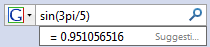Search IntMath
Close

# Google calculator - handy, but not always correct

By Murray Bourne, 27 Aug 2008

Have you ever played with Google's nifty calculator?

Just go to Google and type something like the following into the search field:

4 + 5

The answer will come back at the top of the search results.

What else can it do? Well, lots, actually.

Try these:

• Raise to a power - Example: 5^2
• Square root - Example: sqrt(34.7)
• Trigonometric functions - Example: sin(3pi/4) [It assumes the angle is in radians]
• Counting (in the study of probability) - Example: If you need to know the number of ways 5 objects can be chosen from 14 objects, put: 14 choose 5

There are more examples and information here: Calculator.

BTW, you may not need to actually go to the site. If you have Google selected as the search in Firefox, the answer will appear as a "suggestion" and you don't even need to leave your current page:## Large Numbers and Calculators

Most hand-held calculators are limited when it comes to large numbers. This is a problem if you want to find the factorial of some large-ish number. For example, an old 8-digit calculator I have here can go up to 69! only (the answer is 1.71122 × 1098). [This answer is almost equal to the number "googol" (10100), which was the number that inspired the companies name.]

Now, I can do up to 170! (The answer is 7.25741562 × 10306). After that, it falls over. But hey, not bad.

## Calculator Mistakes

Now, what about those mistakes I was talking about? The following article from CNET.com writer Steven Shankland shows that when the numbers are huge, the calculator could be off a bit (or a lot).

I tend to agree with Shankland. They should put effort into getting this right, with the amount of resources at their disposal you'd think they could...

UPDATE (Dec 2018): Google's phone calculator app appears to have solved most of the issues mentioned by Shankland's (old now) article.

### Comment Preview

HTML: You can use simple tags like <b>, <a href="...">, etc.

To enter math, you can can either:

1. Use simple calculator-like input in the following format (surround your math in backticks, or qq on tablet or phone):
a^2 = sqrt(b^2 + c^2)
(See more on ASCIIMath syntax); or
2. Use simple LaTeX in the following format. Surround your math with $$ and $$.
$$\int g dx = \sqrt{\frac{a}{b}}$$
(This is standard simple LaTeX.)

NOTE: You can mix both types of math entry in your comment.